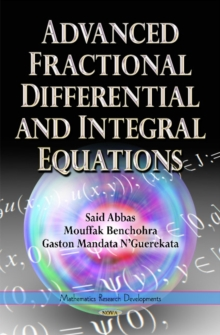Supporting your high street Find out how »
• My AccountAdvanced Fractional Differential & Integral Equations Hardback

Description

Fractional calculus deals with extensions of derivatives and integrals to non-integer orders.

It represents a powerful tool in applied mathematics to study a myriad of problems from different fields of science and engineering, with many break-through results found in mathematical physics, finance, hydrology, biophysics, thermodynamics, control theory, statistical mechanics, astrophysics, cosmology and bioengineering.

This book is devoted to the existence and uniqueness of solutions and some Ulam's type stability concepts for various classes of functional differential and integral equations of fractional order.

Some equations present delay which may be finite, infinite or state-dependent.

Others are subject to multiple time delay effect. The tools used include classical fixed point theorems.

Other tools are based on the measure of non-compactness together with appropriates fixed point theorems.

Each chapter concludes with a section devoted to notes and bibliographical remarks and all the presented results are illustrated by examples.

The content of the book is new and complements the existing literature in Fractional Calculus.

It is useful for researchers and graduate students for research, seminars and advanced graduate courses, in pure and applied mathematics, engineering, biology and other applied sciences.

Information

• Format: Hardback
• Pages: 414 pages
• Publisher: Nova Science Publishers Inc
• Publication Date:
• Category: Differential calculus & equations
• ISBN: 9781634631099

£176.99

£141.99

on all orders

Pick up orders

from local bookshops